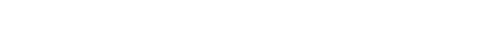glMultMatrix.3glOpenGL man pages

```

```

## Name

```  glMultMatrixd, glMultMatrixf - multiply the current matrix by	an arbitrary
matrix

```

## C Specification

```  void glMultMatrixd( const GLdouble *m	)
void glMultMatrixf( const GLfloat *m )

```

## Parameters

```
m  Specifies a pointer a to 4x4 matrix stored	in column-major	order as
sixteen consecutive values.

```

## Description

```  glMultMatrix multiplies the current matrix with the one specified in m.
That is, if M	is the current matrix and T is the matrix passed to
glMultMatrix,	then M is replaced with	M · T.

The current matrix is	the projection matrix, modelview matrix, or texture
matrix, determined by	the current matrix mode	(see glMatrixMode).

m points to a	4x4 matrix of single- or double-precision floating-point
values stored	in column-major	order.	That is, the matrix is stored as

|a0  a4  a8   a12|
|		       |
|a1  a5  a9   a13|
|		       |
|a2  a6  a10  a14|
|		       |
|a3  a7  a11  a15|

```

## Errors

```  GL_INVALID_OPERATION is generated if glMultMatrix is executed between the
execution of glBegin and the corresponding execution of glEnd.

```

## Associated Gets

```  glGet	with argument GL_MATRIX_MODE
glGet	with argument GL_MODELVIEW_MATRIX
glGet	with argument GL_PROJECTION_MATRIX
glGet	with argument GL_TEXTURE_MATRIX

```

```  glMatrixMode,	glLoadIdentity,	glLoadMatrix, glPushMatrix